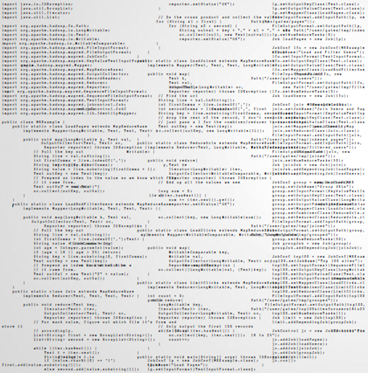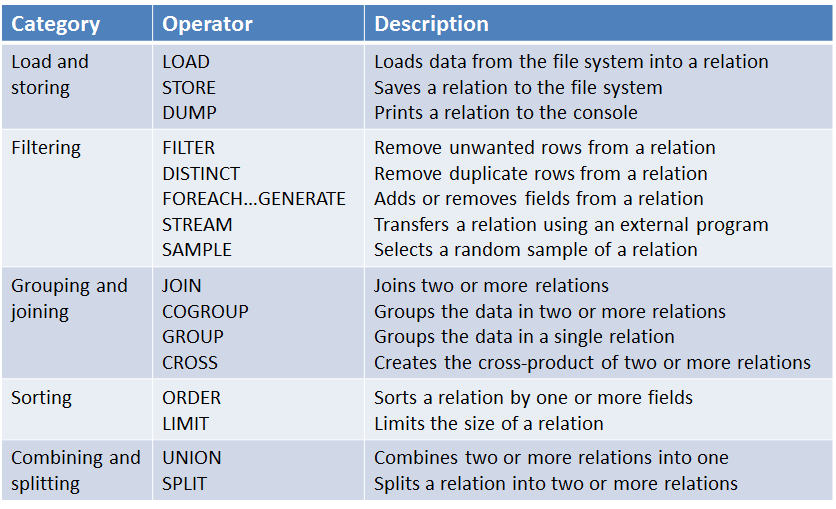## 常用广告系统开源工具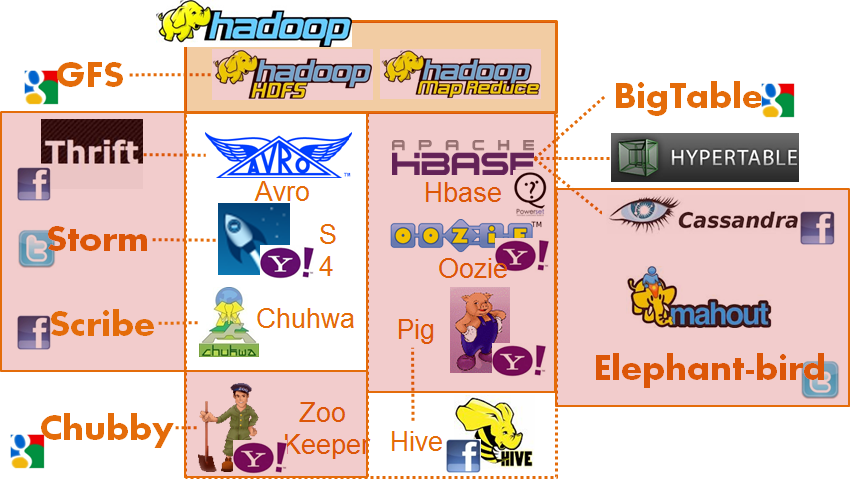• HBASE（列存储的NOSQL数据库，类似的有BigTable，HyperTable，Cassandra）
• Pig: 一种脚本语言
• Elephant-bird：将二进制文件转为 pig 可处理的文本文件
• Hive: 一种脚本语言
• mahout: 分布式机器学习

• ZooKeeper/Chubby: 分布式环境下解决一致性问题
• Avro/Thrift: 分布式环境下跨语言的通信问题
• S4/Storm: 流式计算平台
• Chuhwa/Scribe/Flume: data highway, 分布式日志收集工具，并送到其他平台

## 流量预测

1）售前指导指的是在展示合约广告系统中，由于要约定曝光总数，事先尽可能准确地预测个人群标签的流量非常重要。因为如果流量低估，会出现资源售卖量不足的情形；而如果流量严重高估，则会出现一部分合约不能达成的状况。

2）在线流量分配指的是在展示量合约广告系统中，由于合约之间在人群的选择上会有很多的交集，因此一次的曝光往往会满足多个合约的要求，这时候就需要在多个合约之间进行分配，目的是达到整体满足所有合约的目的。这也是下面要详细探讨的在线流量分配的问题。

3）出价指导是竞价广告中的内容，在竞价广告中，没有了量的保证，广告主往往需要根据自己预计的出价先了解一下可能获得多少流量，以判断自己的出价是否合理。与前面在合约广告中的应用不同，这里还多了出价这一因素。

## 在线分配

### 问题建模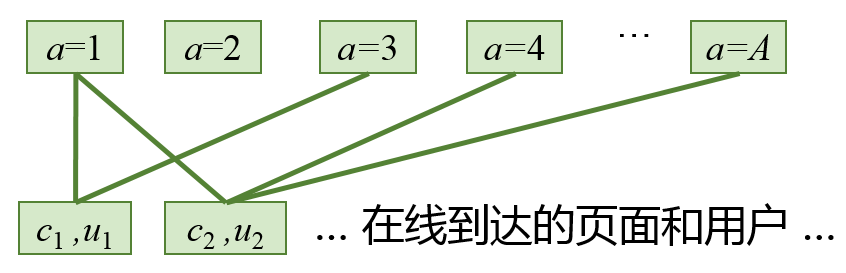GD 问题

\begin{align*} &\max \quad C\\ &\begin{array}\\ s.t. &\sum_{a \in \Gamma(i)} x_{ia} \le 1 &\forall i \in I\\ &\sum_{i \in \Gamma(a)} s_i x_{ia} \ge d_a &\forall a \in A\\ &x_{ia} \ge 0 &\forall (i,a) \in E \end{array} \end{align*}

$C$ 是一个常数，指的是总收益
$I$、$A$、$E$ 上面已经提到，分别表示供给点集合，需求点集合，边的集合
$\Gamma(i)$ 表示与供给节点 $i$ 连接的所有需求节点的集合
$x_{i,a}$ 表示供给节点 $i$ 分配给需求节点 $a$ 的流量的比例
$\Gamma(a)$ 表示所有与需求节点 $a$ 连接的供给节点 $i$ 的集合
$s_i$ 表示供给节点 $i$ 的总流量
$d_a$ 表示需求节点 $a$ 的展示量需求

\begin{align*} &\max_{(i,a) \in E} \quad q_{ia} s_i x_{ia}\\ &\begin{array}\\ s.t. &\sum_{a \in \Gamma(i)} x_{ia} \le 1 & \forall i \in I\\ &\sum_{i \in \Gamma(a)} q_{ia}s_i x_{ia} \le d_a & \forall a \in A\\ &x_{i,a} \ge 0 &\forall (i,a) \in E \end{array} \end{align*}

$q_{ia}$ 表示需求节点(广告主) $a$ 对供给节点（某个人群标签） $i$ 的出价
$d_a$ 表示则表示广告主 $a$ 的总预算

### 问题求解

#### 启发式分配方案 HWM

HWM 分配算法有两个关键点：
1）根据历史流量确定每个广告合约资源的稀缺程度，通过可满足各合约的供给节点总流量的升序排列进而得到分配优先级
2）根据优先级确定每个广告合约的分配比例

## 合约广告系统主要模块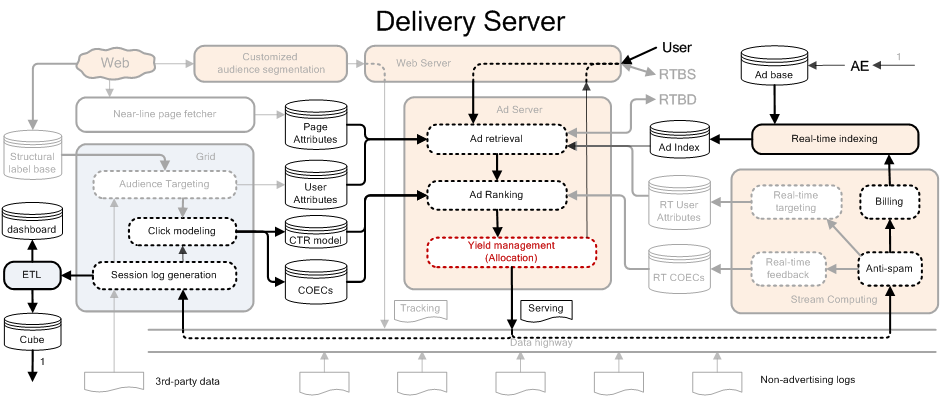Yield management（Allocation）：广告分配的问题

Billing 和 Anti-spam：实时计算部分，用于计价和防作弊，任何广告系统都有

1. HDFS：一个高可靠性, 高效率的分布式文件系统
2. MapReduce: 一个海量数据处理的编程框架

### HDFS

HDFS的架构如下：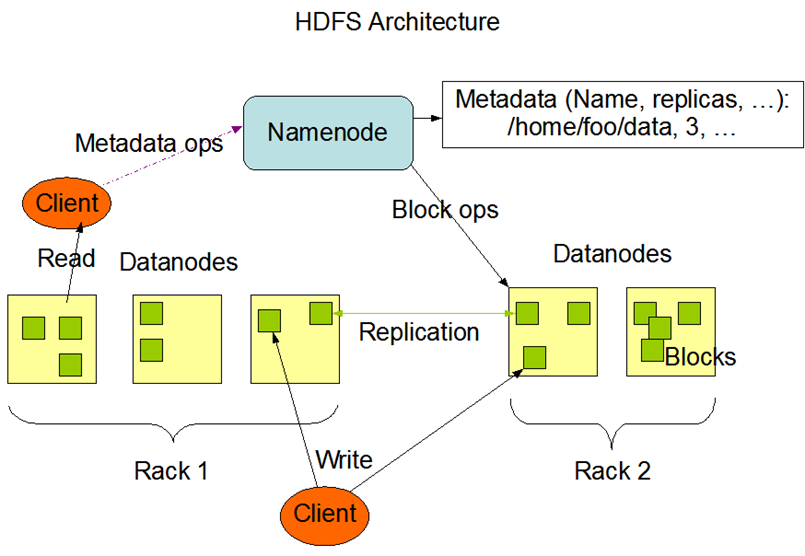HDFS中主要有 Namenode 和 Datanodes 两种角色，其中 Namenode 存储的的是 metadata，其包含的信息是组成文件的各个block存储在哪个 Datanode 上，而 DataNodes 是真正存储文件数据的地方，并且为了达到高可用的效果，文件的一个block会以多个replication的方式存在多个Datanode上。

### MapReduce

MapReduce 是一个分布式计算框架，整个过程包括一个Map过程和一个Reduce过程。相比于MPI,Map 过程处理之间的独立性使得整个系统的可靠性大为提高；并且分布式操作和容错机制由系统实现, 应用级编程非常简单。

MapReduce的计算流程非常类似于简单的Unix pipe

MapReduce中进程间通信的时间只能是在map和reduce间的 shuffle & sort 过程，该过程主要是将经过map操作后 key 相同的那些记录聚合到一起，已进行后面的reduce操作。其过程如下图示：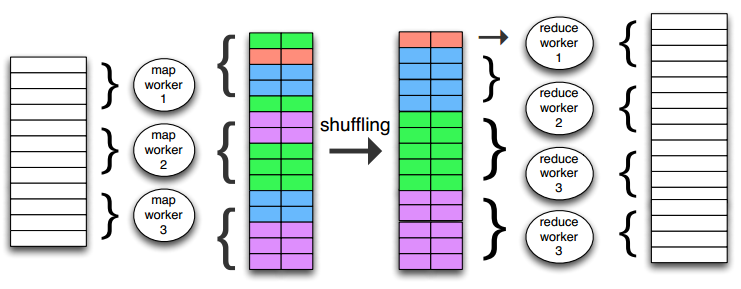### MapReduce 与 分布式机器学习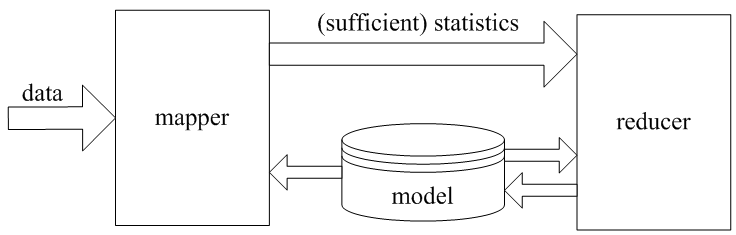### MapReduce 的多种实现方式

MapReduce提供了多样的编程接口，除了上面介绍的直接通过 Java 写MapReduce程序外；通过 Streaming 可以利用标准输入输出模拟以上 pipeline；而通过Pig只需关注数据逻辑，无须考虑M/R实现

Hadoop 的 Streaming 模拟 Pipe 方式执行Map/Reduce Job, 并利用标准输入/输出调度数据；开发者可以使用任何编程语言实现map和reduce过程, 只需要从标准输入读入数据, 并将处理结果打印到标准输出.